# 555 Timer

The 555 timer is a very popular integrated circuit. It is often used in timers, pulse generation and oscillator applications.

### Astable

When the 555 timer is in astable mode, The timer will generate rectangular pulses with a specific frequency. An astable circuit can be connected as in the image below.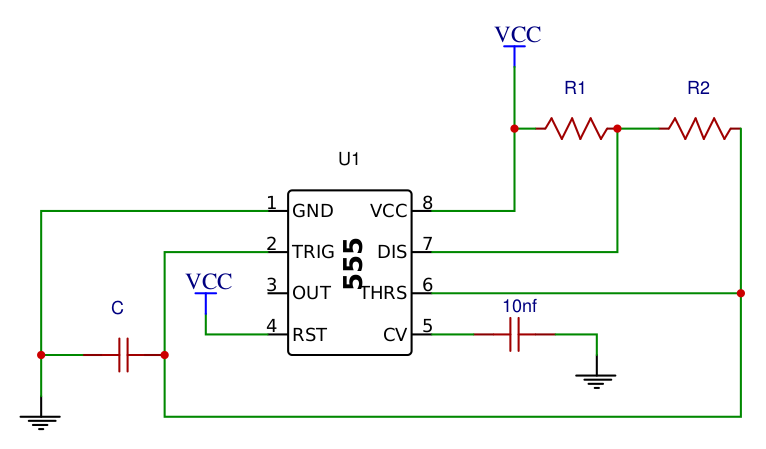#### Formulas

The following formulas can be used to calculate the frequency, period, duty cycle, high time and low time of the 555 timer in astable mode.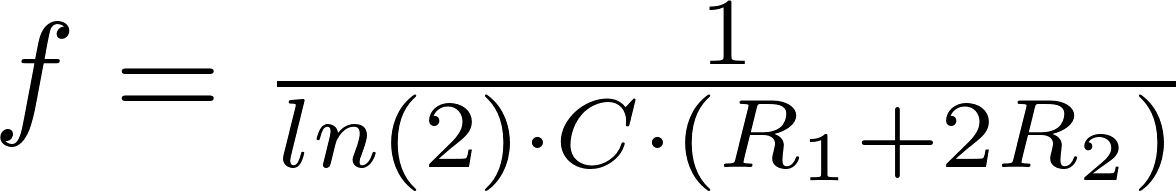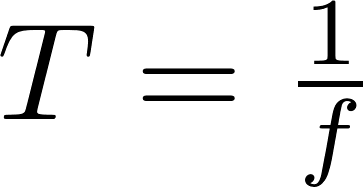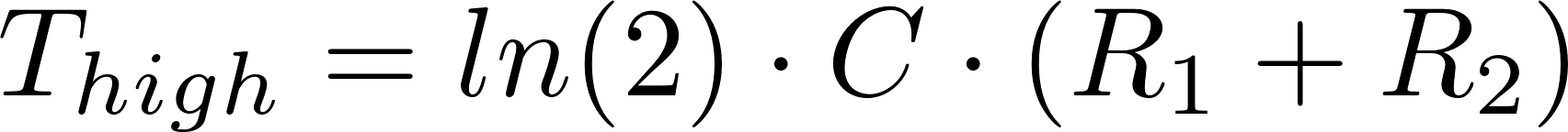f is the symbol for frequency and is measured in hertz (Hz).
C is the symbol for capacitance and is measured in farad (F).
R is the symbol for resistance and is measured in ohm (Ω).
T is the symbol for period and is measured in seconds (s).
DC is the duty cycle and is a percentage

#### Calculator

Enter the capacitance and resistances to calculate all other values.

### Monostable

When the 555 timer is in monostable mode, the generated pulse ends when the voltage on the capacitor is 2/3 of the supply voltage. The length of this pulse can be changed by changing the values of the resistor and capacitor. A monostable circuit is connected as in the image below.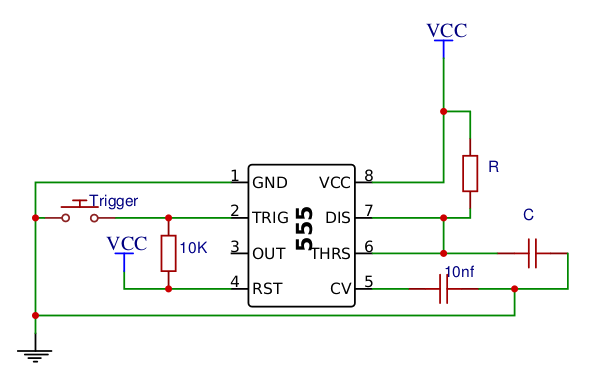#### Formulas

You can use the following formulas to calculate the capacitance, resistance, and output pulse width.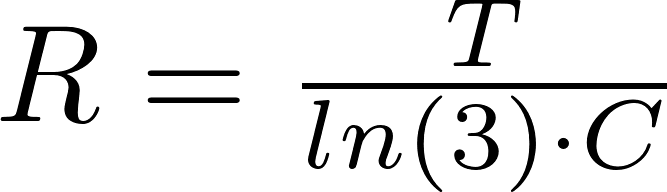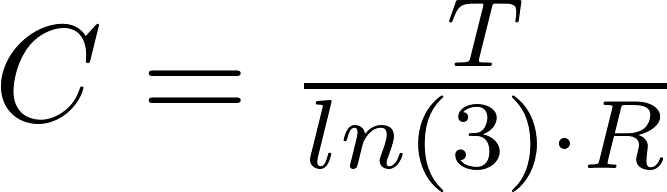T is the symbol for period and is measured in seconds (s).
R is the symbol for resistance and is measured in ohm (Ω).
C is the symbol for capacitance and is measured in farad (F).

#### Calculator

Enter two values to calculate the third.